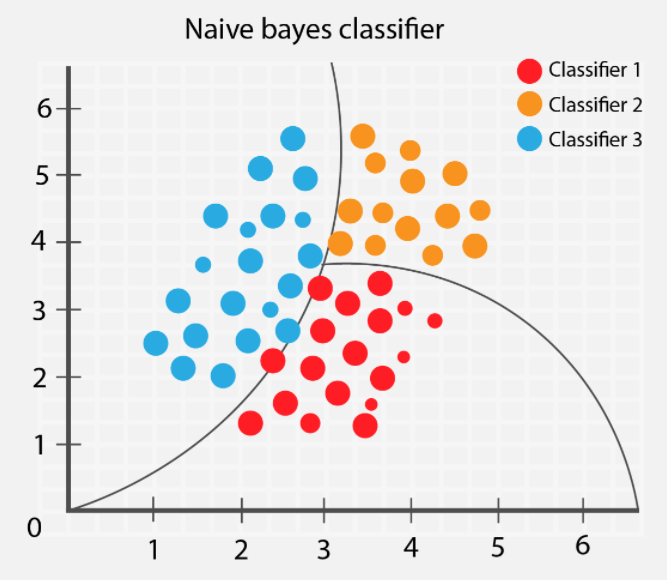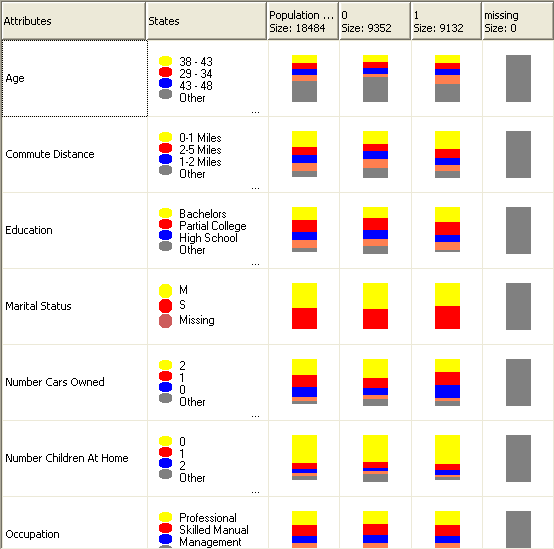EC Data MIning Handbok
Share
Explore
Algoritmer

# Naive Bayes (Classification)Naive Bayes (Classification)The Naive Bayes algorithm calculates the probability of every state of each input column, given each possible state of the predictable column.Here, the Naive Bayes Viewer lists each input column in the dataset, and shows how the states of each column are distributed, given each state of the predictable column.
Usage:
You would use this view of the model to identify the input columns that are important for differentiating between states of the predictable column.
For example, in the row for Commute Distance shown here, the distribution of input values is visibly different for buyers vs. non-buyers. What this tells you is that the input, Commute Distance = 0-1 miles, is a potential predictor.

The viewer also provides values for the distributions, so you can see that for customers who commute from one to two miles to work, the probability of them buying a bike is 0.387, and the probability that they will not buy a bike is 0.287. In this example, the algorithm uses the numeric information, derived from customer characteristics (such as commute distance), to predict whether a customer will buy a bike.

Real time Prediction: Naive Bayes is an eager learning classifier and it is sure fast. Thus, it could be used for making predictions in real time.

Multi class Prediction: This algorithm is also well known for multi class prediction feature. Here we can predict the probability of multiple classes of target variable.

Text classification/ Spam Filtering/ Sentiment Analysis: Naive Bayes classifiers mostly used in text classification (due to better result in multi class problems and independence rule) have higher success rate as compared to other algorithms. As a result, it is widely used in Spam filtering (identify spam e-mail) and Sentiment Analysis (in social media analysis, to identify positive and negative customer sentiments)

Recommendation System: Naive Bayes Classifier and together builds a Recommendation System that uses machine learning and data mining techniques to filter unseen information and predict whether a user would like a given resource or not

The requirements for a Naive Bayes model are as follows:
A single key column Each model must contain one numeric or text column that uniquely identifies each record. Compound keys are not allowed.

Input columns In a Naive Bayes model, all columns must be either discrete, or the values must have been binned. For information about how to discretize (bin) columns, see .

Variables must be independent. For a Naive Bayes model, it is also important to ensure that the input attributes are independent of each other. This is particularly important when you use the model for prediction. If you use two columns of data that are already closely related, the effect would be to multiply the influence of those columns, which can obscure other factors that influence the outcome.

Conversely, the ability of the algorithm to identify correlations among variables is useful when you are exploring a model or dataset, to identify relationships among inputs.

At least one predictable column The predictable attribute must contain discrete or discretized values.

The values of the predictable column can be treated as inputs. This practice can be useful when you are exploring a new dataset, to find relationships among the columns.